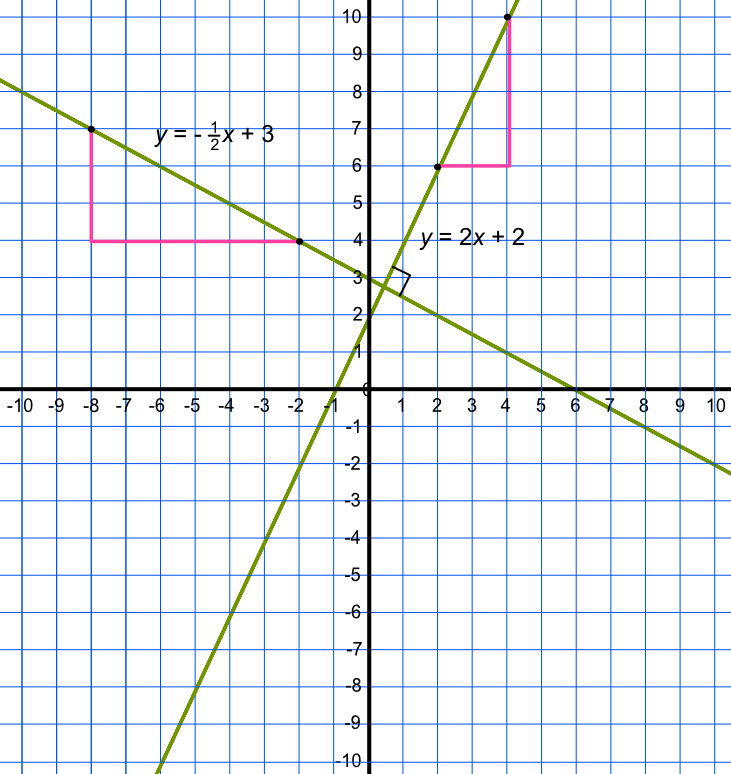Perpendicular Lines

# Perpendicular Lines

GCSE(H),

Perpendicular lines exist at right angles to each other.

Two perpendicular lines are shown on the graph below. The line of one graph is given by y = 2x + 2, and the other by y = -frac(1)(2)x + 3.

Lines are perpendicular if the gradients of the two lines multiplied together = -1.## Examples

1. What is the equation of the line that is perpendicular to y = 3x -3 that also passes through (0, 5)?

Answer: y = -frac(1)(3)x + 5

The gradient of the original line is 3. The gradient of the perpendicular line = - frac(1)(3) (reciprocal of the original gradient x -1).

The intercept of the line is given by the fact that it passes through (0, 5).

2. Find the equation of the line perpendicular to y = frac(1)(2)x - 3 that passes through (4, 4).

Answer: y = -2x + 12

The gradient of the second line is given by - 1 / frac(1)(2) = -2.

The lines passes through the point (4, 4): 4 = -2 x 4 + c, where c is the intercept = 12.

The equation of the line is y = -2x + 12.